# meshz

Mesh surface plot with curtain

•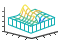## Syntax

``meshz(X,Y,Z)``
``meshz(X,Y,Z,C)``
``meshz(Z)``
``meshz(Z,C)``
``meshz(___,Name,Value)``
``meshz(ax,___)``
``s = meshz(___)``

## Description

example

````meshz(X,Y,Z)` creates a mesh plot with a curtain around it. A mesh plot is a three-dimensional surface that has solid edge colors and no face colors. The function plots the values in matrix `Z` as heights above a grid in the x-y plane defined by `X` and `Y`. The edge colors vary according to the heights specified by `Z`.```

example

````meshz(X,Y,Z,C)` additionally specifies the color of the edges.```
````meshz(Z)` creates a mesh plot with a curtain, and uses the column and row indices of the elements in `Z` as the x- and y-coordinates.```
````meshz(Z,C)` specifies the color of the edges.```
````meshz(___,Name,Value)` specifies additional options for the `meshz` plot using one or more name-value pair arguments. Specify the options after all other input arguments. For a list of properties, see Surface Properties.```
````meshz(ax,___)` plots into the axes specified by `ax` instead of the current axes. Specify the axes as the first input argument.```
````s = meshz(___)` returns the chart surface object. Use `s` to modify the mesh plot after it is created. For a list of properties, see Surface Properties.```

## Examples

collapse all

Create three matrices of the same size. Then plot them as a mesh plot with a curtain. The mesh plot uses `Z` for both height and color.

```[X,Y] = meshgrid(-3:.125:3); Z = peaks(X,Y); meshz(X,Y,Z)```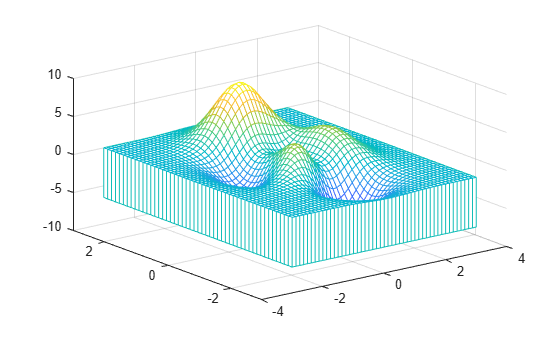Specify the colors for a mesh plot and surrounding curtain by including a fourth matrix input, `C`. The mesh plot uses `Z` for height and `C` for color. Specify the colors using a colormap, which uses single numbers to stand for colors on a spectrum. When you use a colormap, `C` is the same size as `Z`. Add a color bar to the graph to show how the data values in `C` correspond to the colors in the colormap.

```[X,Y] = meshgrid(-3:.125:3); Z = peaks(X,Y); C = gradient(Z); meshz(X,Y,Z,C) colorbar```Create a mesh plot with a curtain around it. To allow further modifications, assign the surface object to the variable `s`.

```[X,Y] = meshgrid(-5:.5:5); Z = Y.*sin(X) - X.*cos(Y); s = meshz(X,Y,Z)``````s = Surface (meshz) with properties: EdgeColor: 'flat' LineStyle: '-' FaceColor: [1 1 1] FaceLighting: 'none' FaceAlpha: 1 XData: [25x25 double] YData: [25x25 double] ZData: [25x25 double] CData: [25x25 double] Use GET to show all properties ```

Use `s` to access and modify properties of the mesh plot after it is created. For example, change the color of the mesh plot edges and surrounding curtain by setting the `EdgeColor` property.

`s.EdgeColor = 'b';`## Input Arguments

collapse all

x-coordinates, specified as a matrix the same size as `Z`, or as a vector with length `n`, where `[m,n] = size(Z)`. If you do not specify values for `X` and `Y`, `meshz` uses the vectors `(1:n)` and `(1:m)`.

When `X` is a matrix, the values must be strictly increasing or decreasing along one dimension and remain constant along the other dimension. The dimension that varies must be the opposite of the dimension that varies in `Y`. You can use the `meshgrid` function to create `X` and `Y` matrices.

When `X` is a vector, the values must be strictly increasing or decreasing.

The `XData` property of the surface object stores the x-coordinates.

Example: `X = 1:10`

Example: `X = [1 2 3; 1 2 3; 1 2 3]`

Example: `[X,Y] = meshgrid(-5:0.5:5)`

Data Types: `single` | `double` | `int8` | `int16` | `int32` | `int64` | `uint8` | `uint16` | `uint32` | `uint64` | `categorical`

y-coordinates, specified as a matrix the same size as `Z` or as a vector with length `m`, where `[m,n] = size(Z)`. If you do not specify values for `X` and `Y`, `meshz` uses the vectors `(1:n)` and `(1:m)`.

When `Y` is a matrix, the values must be strictly increasing or decreasing along one dimension and remain constant along the other dimension. The dimension that varies must be the opposite of the dimension that varies in `X`. You can use the `meshgrid` function to create `X` and `Y` matrices.

When `Y` is a vector, the values must be strictly increasing or decreasing.

The `YData` property of the surface object stores the y-coordinates.

Example: `Y = 1:10`

Example: `Y = [1 1 1; 2 2 2; 3 3 3]`

Example: `[X,Y] = meshgrid(-5:0.5:5)`

Data Types: `single` | `double` | `int8` | `int16` | `int32` | `int64` | `uint8` | `uint16` | `uint32` | `uint64` | `categorical`

z-coordinates, specified as a matrix. `Z` must have at least two rows and two columns.

`Z` specifies the height of the mesh plot at each x-y coordinate. If you do not specify the colors, then `Z` also specifies the mesh edge colors.

The `ZData` property of the surface object stores the z-coordinates.

Example: `Z = [1 2 3; 4 5 6]`

Example: `Z = sin(x) + cos(y)`

Data Types: `single` | `double` | `int8` | `int16` | `int32` | `int64` | `uint8` | `uint16` | `uint32` | `uint64` | `categorical`

Color array, specified as an m-by-n matrix of colormap indices, where `Z` is `m`-by-`n`. For each grid point on the mesh surface, `C` indicates a color in the colormap. The `CDataMapping` property of the surface object controls how the values in `C` correspond to colors in the colormap.

The `CData` property of the surface object stores the color array. For additional control over the surface coloring, use the `FaceColor` and `EdgeColor` properties.

Axes to plot in, specified as an `axes` object. If you do not specify the axes, then `meshz` plots into the current axes.

### Name-Value Arguments

Specify optional pairs of arguments as `Name1=Value1,...,NameN=ValueN`, where `Name` is the argument name and `Value` is the corresponding value. Name-value arguments must appear after other arguments, but the order of the pairs does not matter.

Before R2021a, use commas to separate each name and value, and enclose `Name` in quotes.

Example: `meshz(X,Y,Z,'EdgeColor','red')` creates the mesh with red lines.

Note

The properties listed here are only a subset. For a full list, see Surface Properties.

Edges to display, specified as `'both'`, `'row'`, or `'column'`.

Edge line color, specified as one of the values listed here. The default color of `[0 0 0]` corresponds to black edges.

ValueDescription
`'none'`Do not draw the edges.
`'flat'`

Use a different color for each edge based on the values in the `CData` property. First you must specify the `CData` property as a matrix the same size as `ZData`. The color value at the first vertex of each face (in the positive x and y directions) determines the color for the adjacent edges. You cannot use this value when the `EdgeAlpha` property is set to `'interp'`.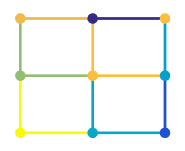`'interp'`

Use interpolated coloring for each edge based on the values in the `CData` property. First you must specify the `CData` property as a matrix the same size as `ZData`. The color varies across each edge by linearly interpolating the color values at the vertices. You cannot use this value when the `EdgeAlpha` property is set to `'flat'`.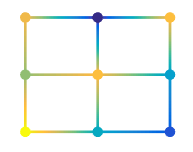RGB triplet, hexadecimal color code, or color name

Use the specified color for all the edges. This option does not use the color values in the `CData` property.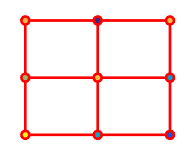RGB triplets and hexadecimal color codes are useful for specifying custom colors.

• An RGB triplet is a three-element row vector whose elements specify the intensities of the red, green, and blue components of the color. The intensities must be in the range `[0,1]`; for example,``` [0.4 0.6 0.7]```.

• A hexadecimal color code is a character vector or a string scalar that starts with a hash symbol (`#`) followed by three or six hexadecimal digits, which can range from `0` to `F`. The values are not case sensitive. Thus, the color codes `"#FF8800"`, `"#ff8800"`, `"#F80"`, and `"#f80"` are equivalent.

Alternatively, you can specify some common colors by name. This table lists the named color options, the equivalent RGB triplets, and hexadecimal color codes.

Color NameShort NameRGB TripletHexadecimal Color CodeAppearance
`"red"``"r"``[1 0 0]``"#FF0000"``"green"``"g"``[0 1 0]``"#00FF00"``"blue"``"b"``[0 0 1]``"#0000FF"``"cyan"` `"c"``[0 1 1]``"#00FFFF"``"magenta"``"m"``[1 0 1]``"#FF00FF"``"yellow"``"y"``[1 1 0]``"#FFFF00"``"black"``"k"``[0 0 0]``"#000000"``"white"``"w"``[1 1 1]``"#FFFFFF"`Here are the RGB triplets and hexadecimal color codes for the default colors MATLAB® uses in many types of plots.

`[0 0.4470 0.7410]``"#0072BD"``[0.8500 0.3250 0.0980]``"#D95319"``[0.9290 0.6940 0.1250]``"#EDB120"``[0.4940 0.1840 0.5560]``"#7E2F8E"``[0.4660 0.6740 0.1880]``"#77AC30"``[0.3010 0.7450 0.9330]``"#4DBEEE"``[0.6350 0.0780 0.1840]``"#A2142F"`Face color, specified as one of the values in this table.

ValueDescription
`'flat'`

Use a different color for each face based on the values in the `CData` property. First you must specify the `CData` property as a matrix the same size as `ZData`. The color value at the first vertex of each face (in the positive x and y directions) determines the color for the entire face. You cannot use this value when the `FaceAlpha` property is set to `'interp'`.`'interp'`

Use interpolated coloring for each face based on the values in the `CData` property. First you must specify the `CData` property as a matrix the same size as `ZData`. The color varies across each face by interpolating the color values at the vertices. You cannot use this value when the `FaceAlpha` property is set to `'flat'`.RGB triplet, hexadecimal color code, or color name

Use the specified color for all the faces. This option does not use the color values in the `CData` property.`'texturemap'`Transform the color data in `CData` so that it conforms to the surface.
`'none'`Do not draw the faces.

RGB triplets and hexadecimal color codes are useful for specifying custom colors.

• An RGB triplet is a three-element row vector whose elements specify the intensities of the red, green, and blue components of the color. The intensities must be in the range `[0,1]`; for example,``` [0.4 0.6 0.7]```.

• A hexadecimal color code is a character vector or a string scalar that starts with a hash symbol (`#`) followed by three or six hexadecimal digits, which can range from `0` to `F`. The values are not case sensitive. Thus, the color codes `"#FF8800"`, `"#ff8800"`, `"#F80"`, and `"#f80"` are equivalent.

Alternatively, you can specify some common colors by name. This table lists the named color options, the equivalent RGB triplets, and hexadecimal color codes.

Color NameShort NameRGB TripletHexadecimal Color CodeAppearance
`"red"``"r"``[1 0 0]``"#FF0000"``"green"``"g"``[0 1 0]``"#00FF00"``"blue"``"b"``[0 0 1]``"#0000FF"``"cyan"` `"c"``[0 1 1]``"#00FFFF"``"magenta"``"m"``[1 0 1]``"#FF00FF"``"yellow"``"y"``[1 1 0]``"#FFFF00"``"black"``"k"``[0 0 0]``"#000000"``"white"``"w"``[1 1 1]``"#FFFFFF"`Here are the RGB triplets and hexadecimal color codes for the default colors MATLAB uses in many types of plots.

`[0 0.4470 0.7410]``"#0072BD"``[0.8500 0.3250 0.0980]``"#D95319"``[0.9290 0.6940 0.1250]``"#EDB120"``[0.4940 0.1840 0.5560]``"#7E2F8E"``[0.4660 0.6740 0.1880]``"#77AC30"``[0.3010 0.7450 0.9330]``"#4DBEEE"``[0.6350 0.0780 0.1840]``"#A2142F"`Edge transparency, specified as one of these values:

• Scalar in range `[0,1]` — Use uniform transparency across all of the edges. A value of `1` is fully opaque and `0` is completely transparent. Values between `0` and `1` are semitransparent. This option does not use the transparency values in the `AlphaData` property.

• `'flat'` — Use a different transparency for each edge based on the values in the `AlphaData` property. First you must specify the `AlphaData` property as a matrix the same size as the `ZData` property. The transparency value at the first vertex determines the transparency for the entire edge. The `EdgeColor` property also must be set to `'flat'`.

• `'interp'` — Use interpolated transparency for each edge based on the values in `AlphaData` property. First you must specify the `AlphaData` property as a matrix the same size as the `ZData` property. The transparency varies across each edge by interpolating the values at the vertices. The `EdgeColor` property also must be set to `'interp'`.

Line style, specified as one of the options listed in this table.

Line StyleDescriptionResulting Line
`"-"`Solid line`"--"`Dashed line`":"`Dotted line`"-."`Dash-dotted line`"none"`No lineNo line

Line width, specified as a positive value in points, where 1 point = 1/72 of an inch. If the line has markers, then the line width also affects the marker edges.

The line width cannot be thinner than the width of a pixel. If you set the line width to a value that is less than the width of a pixel on your system, the line displays as one pixel wide.

## Version History

Introduced before R2006a